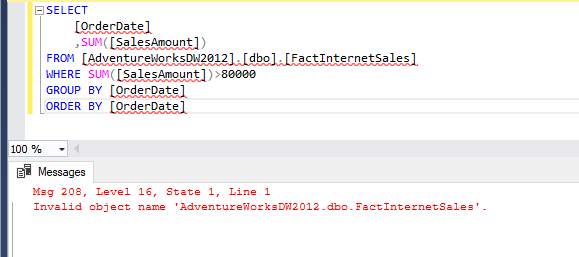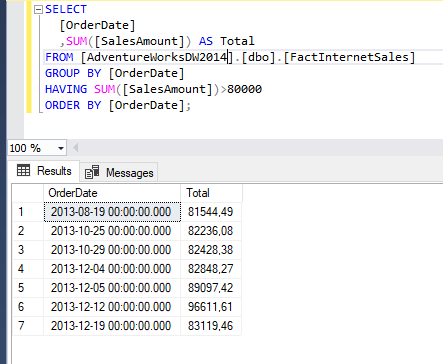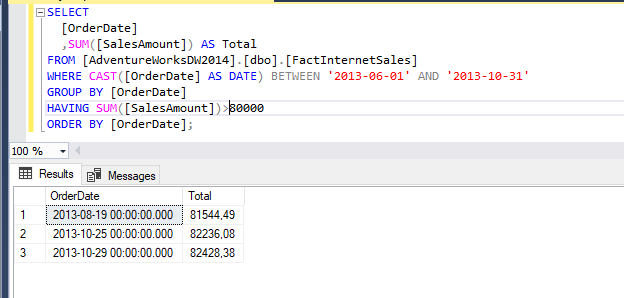• 17. 11. 2019
• Jan Zedníček - Data & Finance
• 0

HAVING clause is a special type of SQL command. It works in a similar way as WHERE clause and we use it to define a condition. There are important differences between the two clauses. Let me explain the differences between SQL HAVING and WHERE.

## Having  in SQL script

1. SELECT
2. FROM
3. WHERE
4. GROUP BY
5. HAVING
6. ORDER BY

Why do we have 2 clauses to define conditions? The answer can be found in article about logical processing of SQL query. We can see that the processing order of clauses in the script is following:

1. FROM,
2. WHERE,
3. GROUP BY,
4. HAVING,
5. SELECT,
6. ORDER BY

It is clear from what is mentioned above that e.g. aggregate operations (GROUP BY) are processed after WHERE clause is completed. Let’s think about Where in SQL script would we type requirement based on aggregation SUM(metric)>0? We would have to process the requirement twice (through nested query, CTE etc.). Luckily, this will not be needed and we can use clause HAVING for similar situations.

Summary:

• In WHERE are written conditions which operate with data in original (non-aggregated) form
• A Condition within Aggregation is being entered in HAVING

HAVING is used mainly to assign condition based on the aggregate function

## Syntax

`SELECT [Column 1],[Column 2], SUM([Column 3]) AS TotalFROM TableWHERE Condition 1...Condition nGROUP BY [Column 1],[Column 2]HAVING SUM([Column 3]) <operator> Condition`

## HAVING Example

Lets’s have the table [FactInternetSales]. We will work with only 2 fields:

• Date [OrderDate]
• Amount [SalesAmount]As in the task in the article about GROUP BY we will want to aggregate the amount according to dates. The result will be sorted in ascending order this time. We will also want to display only dates where the sum of all sales in total is higher than 80 000. We will use:

• aggregate function SUM() in SELECT clause
• GROUP BY
• HAVING
• ORDER BY

### Commutation of SQL HAVING vs WHERE – Let us take a look at what happens when we commutate the clauses

What will happen when we write requirement based on aggregate function into WHERE:

`SELECT `
`  [OrderDate]`
`  ,SUM([SalesAmount]) AS Total`
`FROM [AdventureWorksDW2012].[dbo].[FactInternetSales]`
`WHERE SUM([SalesAmount]) > 80000`
`GROUP BY [OrderDate]`
`ORDER BY [OrderDate]`

the result is an error:0

### How to Write SQL script with HAVING Correctly?

We will use HAVING instead of WHERE:

`SELECT `
`  [OrderDate]`
`  ,SUM([SalesAmount]) AS Total`
`FROM [AdventureWorksDW2012].[dbo].[FactInternetSales]`
`GROUP BY [OrderDate]`
`HAVING SUM([SalesAmount])>80000`
`ORDER BY [OrderDate];`

The result is OK:## Combination of SQL HAVING and WHERE

We can obviously combine WHERE and HAVING. Imagine we want only Dates from 2013-06-01 till 2013-10-31 where the sum of sales is higher than 80 000. The condition for date column will be typed into WHERE and condition for aggregation into having as shown on this example:The script was executed successfully without an error. We can see that between 2013-06-01 and 2013-10-31 was 3 days where the sum of the sales is higher than 80 000 USD.

Rate this post### Jan Zedníček - Data & Finance

My name is Jan Zedníček and I work as a freelancer. I have been working as a freelancer for many companies for more than 10 years.
I used to work as a financial controller, analyst and manager at many different companies in field of banking and manufacturing. When I am not at work, I like playing volleyball, chess, doing a workout in the gym and I enjoy tasting of best quality rums.
I am trying to summarize all my knowledge on this website not to forget them and to put them forward to anyone.